Next: Single-particle structures at N=Z=16 Up: Superdeformed bands in S Previous: Introduction

# Theoretical methods

In this paper we use the cranking Hartree-Fock (HF) method with the Skyrme SLy4 interaction . The complete gauge-invariant  term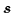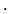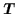-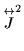has been removed from the Skyrme functional in order to comply with the procedure of adjusting the parameters of this force . We solve the self-consistent HF equations by using the HFODD code (v1.75) [35,36], that employs the Cartesian HO basis. The basis used consists of the lowest M=306 HO states with the oscillator frequencies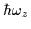=11.46MeV and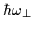=18.01MeV. These parameters correspond to including in the basis up to Nz=14 and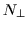=9 HO quanta. As discussed in Ref. , no further basis optimization is necessary, and thus the same unique basis has been used for all calculations presented below. In the calculations, the conservation of parity and signature symmetries has been assumed.

In Ref.  it was shown that by using a much larger HO basis of M=1200, one obtains a perfect agreement (up to 18keV) of the 152Dy binding energies with those calculated using the space-coordinate code of Ref. . At the same time, the M=300 calculations were giving a systematic underbinding of about 5MeV, which, however, was very weakly dependent on the angular frequency or configuration. Here, we repeat similar tests in 32S. At the spherical shape, with M=306 we obtain the total energy of E=-270.000MeV, while a simple one-dimensional coordinate-space code gives E=-270.876MeV. At the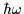=1MeV SD magic configuration of 32S (see below), the M=306 result for the total routhian is R=E-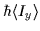=-261.453MeV, while M=1200 (Nz=24 and=15) gives R=-262.124MeV. From these results we conclude that the absolute energies of all nuclei presented in this paper should be shifted down, at all deformations and at all rotational frequencies, by a constant of about 0.8MeV, in order to account for the finite size of the HO basis used in the calculations.

We have also performed the Hartree-Fock-Bogoliubov (HFB) calculations in 32S, by using the HFODD code (v1.79) for the zero-range (density independent) pairing interaction in the particle-particle channel, and the Skyrme SLy4 force in the particle-hole channel. The strength of the pairing interaction has been adjusted to obtain the value of the average pairing gap (at the spherical shape) equal to the one obtained with the help of the three-point mass staggering expression , applied to experimental masses of nuclei adjacent to 32S.

It turns out that the static pairing correlations, calculated within the HFB approximation for such a pairing strength, vanish at the SD shapes. With an artificially increased strength one may, of course, obtain non-zero pairing at the SD band-heads, but the HFB static pairing correlations disappear again very rapidly with increasing spin. Consequently, these calculations show that the proton-proton and the neutron-neutron pairing correlations do not contribute very strongly to the structure of the SD states in 32S, and may possibly affect the results only through dynamic correlations.

The above remarks do not exclude the possibility that, in the nuclei of interest here, strong proton-neutron pairing correlations may take place. To the contrary, in analogy to a suggestion relevant in the A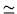60 region , also in 32S we may expect strong proton-neutron pairing correlations at high spins. An approach which would take all these pairing mechanisms simultaneously into account is fairly complicated, and no appropriate tools exist to date to carry out such a program. Moreover, the experimental information about the proton-neutron pairing correlations at high spins should be considered as very limited today. Therefore the results presented below do not include the effect of the pairing interactions. This, as argued above, offers a reasonable approximation, and allows for a rapid overview of all available lowest-energy configurations. Such an analysis should be considered as a sufficient first step towards a more complete future study, given the fact that experimental results on the corresponding high spin effects do not exist at present.Next: Single-particle structures at N=Z=16 Up: Superdeformed bands in S Previous: Introduction
Jacek Dobaczewski
1999-07-27